150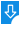10
2585

Fibonacci Spiral Model Description:
Fibonacci Spiral Model is a small simulation that draws a spiral with its growth regulated by the Fibonacci series. Fibonacci Spiral Model's growth parallels the growth of the series itself. In order to produce an uniform distribution in the plane of the spiral, points are separated by the golden angle. Fibonacci Spiral Model is developed using the Java programming language and can be run on Mac OS X, Windows and Linux.Fibonacci Spiral Model: Fibonacci Spiral Model for Mac, Java simulation that allows you to draw Fibonacci series spirals
Publisher: Wolfgang ChristianDownload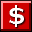Buy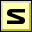Screenshot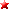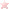Rate it
Category: Math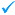Publisher: Wolfgang Christian    More titles >>
Last Updated: 03/05/2019
Requirements: Mac OS X